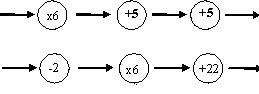#### You may also like### Exploring Wild & Wonderful Number Patterns

EWWNP means Exploring Wild and Wonderful Number Patterns Created by Yourself! Investigate what happens if we create number patterns using some simple rules.### Sending Cards

This challenge asks you to investigate the total number of cards that would be sent if four children send one to all three others. How many would be sent if there were five children? Six?### Dice and Spinner Numbers

If you had any number of ordinary dice, what are the possible ways of making their totals 6? What would the product of the dice be each time?

# Function Machines

##### Age 7 to 11Challenge Level

Well done to all of you who gave a correct solution to this problem. The correct answer to the first part of the question is $33, 48, 38$.

There are several correct answers to the second part of the problem including:Richard from Burlingame School used a systematic approach to help him find the answer to the second part of the problem:

What I did was multiply each number by $2$, then $3$, then $4$, etc till I found a pattern. If you multiply the number by $6$, you come out with a number $10$ less than the answer. I simply added ten, then subtracted $0$ to give me the answer.

Quite a few of you used a really useful technique to help find the solution more efficiently including Marley and Jake from Swarcliffe Primary and Shiv from Mayplace Primary School. Ester sent in a nice solution:

The numbers are CONSECUTIVE going in and HAVE A DIFFERENCE OF $6$ coming out. This tells us that there must be a multiplication by $6$ somewhere.

She also spotted that there was more than one solution:

If $\times 6$ is in the middle circle some solutions could be

$+2, \times 6, -2$

$+1, \times 6, +4$

$-1, \times 6, +16$

If you multiply by six first you have to add or subtract in the last two circles so that the result is $+10$

$\times ,+6,+4$

$\times 6,+11,-1$

You cannot make the $\times 6$ the last thing you do because the numbers coming out are not in the six times table.

As you can see, there are lots of possible solutions using multiply by $6$ as one of the functions, but you can also split multiplying by $6$ into multiplying by $3$ and multiplying by $2$, or as several pupils from the Mount School in York observed, multiplying by $12$ and dividing by $2$.